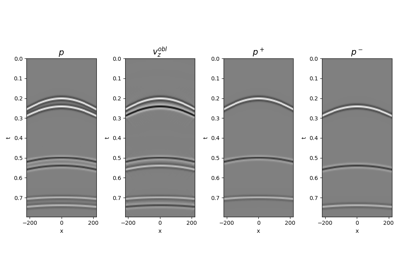# pylops.waveeqprocessing.WavefieldDecomposition#

pylops.waveeqprocessing.WavefieldDecomposition(p, vz, nt, nr, dt, dr, rho, vel, nffts=(None, None, None), critical=100.0, ntaper=10, scaling=1.0, kind='inverse', restriction=None, sptransf=None, solver=<function lsqr>, dottest=False, dtype='complex128', **kwargs_solver)[source]#

Up-down wavefield decomposition.

Apply seismic wavefield decomposition from multi-component (pressure and vertical particle velocity) data. This process is also generally referred to as data-based deghosting.

Parameters
pnp.ndarray

Pressure data of size $$\lbrack n_{r_x} \,(\times n_{r_y}) \times n_t \rbrack$$ (or $$\lbrack n_{r_{x,\text{sub}}} \,(\times n_{r_{y,\text{sub}}}) \times n_t \rbrack$$ in case a restriction operator is provided. Note that $$n_{r_{x,\text{sub}}}$$ (and $$n_{r_{y,\text{sub}}}$$) must agree with the size of the output of this operator.)

vznp.ndarray

Vertical particle velocity data of same size as pressure data

ntint

Number of samples along the time axis

nr

Number of samples along the receiver axis (or axes)

dtfloat

Sampling along the time axis

dr

Sampling along the receiver array (or axes)

rhofloat

Density $$\rho$$ along the receiver array (must be constant)

velfloat

Velocity $$c$$ along the receiver array (must be constant)

nfftstuple, optional

Number of samples along the wavenumber and frequency axes

criticalfloat, optional

Percentage of angles to retain in obliquity factor. For example, if critical=100 only angles below the critical angle $$\frac{f(k_x)}{c}$$ will be retained

ntaperfloat, optional

Number of samples of taper applied to obliquity factor around critical angle

kindstr, optional

Type of separation: inverse (default) or analytical

scalingfloat, optional

Scaling to apply to the operator (see Notes of pylops.waveeqprocessing.wavedecomposition.UpDownComposition2D for more details)

restrictionpylops.LinearOperator, optional

Restriction operator

sptransfpylops.LinearOperator, optional

Sparsifying operator

solverfloat, optional

Function handle of solver to be used if kind='inverse'

dottestbool, optional

Apply dot-test

dtypestr, optional

Type of elements in input array.

**kwargs_solver

Arbitrary keyword arguments for chosen solver

Returns
pupnp.ndarray

Up-going wavefield

pdownnp.ndarray

Down-going wavefield

Raises
KeyError

If kind is neither analytical nor inverse

Notes

Up- and down-going components of seismic data $$p^-(x, t)$$ and $$p^+(x, t)$$ can be estimated from multi-component data $$p(x, t)$$ and $$v_z(x, t)$$ by computing the following expression :

$\begin{split}\begin{bmatrix} \hat{p}^+ \\ \hat{p}^- \end{bmatrix}(k_x, \omega) = \frac{1}{2} \begin{bmatrix} 1 & \frac{\omega \rho}{k_z} \\ 1 & - \frac{\omega \rho}{k_z} \\ \end{bmatrix} \begin{bmatrix} \hat{p} \\ \hat{v}_z \end{bmatrix}(k_x, \omega)\end{split}$

if kind='analytical' or alternatively by solving the equation in ptcpy.waveeqprocessing.UpDownComposition2D as an inverse problem, if kind='inverse'.

The latter approach has several advantages as data regularization can be included as part of the separation process allowing the input data to be aliased. This is obtained by solving the following problem:

$\begin{split}\begin{bmatrix} \mathbf{p} \\ s\mathbf{v_z} \end{bmatrix} = \begin{bmatrix} \mathbf{R}\mathbf{F} & 0 \\ 0 & s\mathbf{R}\mathbf{F} \end{bmatrix} \mathbf{W} \begin{bmatrix} \mathbf{F}^H \mathbf{S} & 0 \\ 0 & \mathbf{F}^H \mathbf{S} \end{bmatrix} \mathbf{p^{\pm}}\end{split}$

where $$\mathbf{R}$$ is a ptcpy.basicoperators.Restriction operator and $$\mathbf{S}$$ is sparsyfing transform operator (e.g., ptcpy.signalprocessing.Radon2D).

1

Wapenaar, K. “Reciprocity properties of one-way propagators”, Geophysics, vol. 63, pp. 1795-1798. 1998.

## Examples using pylops.waveeqprocessing.WavefieldDecomposition#14. Seismic wavefield decomposition

14. Seismic wavefield decomposition